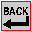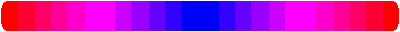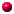CALCULATING CHARTWARP:

Length in inches
Width in inches
Ends per inch
 L W E

Length X Width X Ends = Total inches

 X X x =

Total Yards= Total inches / 36

NOTE : Length of warp must include project(place mat), space between projects(place mats), and waste at both ends of warp to attach to loom.
The number of Ends per inch can be estimated by wraping yarn around a ruler, then counting wraps per inch and dividing by 2.WEFT:

Length in inches
Width in inches
Insertions per inch (inwouven ends)
 L W I

Length X Width X Insertions + 20% = Total inches

 X X x +20% =

Total Yards = Total inches / 36

NOTE : No allowance needs to be made for areas that will not have weft.
Extra weft allowances are estimates and therefore will greatly depend on; the type of weft and warp, the pattern, and the tension.Please Note: The calculations arrived at here are only approximate.
Print-out Form provided on Curl Bros, Textiles Ltd. web Site: www.curlbros.com